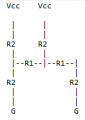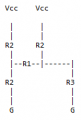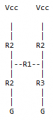# Simple circuit - equivalent impedance - amateur question

#### jduncalf

Joined Apr 21, 2022
3
Hi, i'm currently teaching myself electronics; In doing so i've encountered a circuit which my current grasp of theory is failing to understand. The circuit is derived from a 3bit R2R ladder, bits 1&2 are set high. Here's an ascii pictorial version of the circuit:

Vcc is 5V
R1 is 3.3K, R2 is 6.8KMy usual approach is to reduce the parallel portions of the circuit and simplify, so that I can calculate the total impedance and then the current, then the voltage drops/voltages at each nodes.

The above circuit slightly simplified:And again:So from this point my understanding of theory fails me. I can only arrive at the correct (measured at 7.4K) value by totally ignoring R1, calculating:

(R2 + R2) in parallel with (R2 + R3), i.e.
R4 in parallel with R5, i.e.
13.6K in parallel with 16.9K = 7.54k!

This is the closest I can calculate to the measured value, however of course R1 is missing. I'd greatly appreciate any help and theory corrections.

Regards.

#### StefanZe

Joined Nov 6, 2019
187

#### jduncalf

Joined Apr 21, 2022
3
Thanks, I hadn't heard of that until now. Working on it...

Regards.

#### jduncalf

Joined Apr 21, 2022
3
Thanks, I hadn't heard of that until now. Working on it...

Regards.
Thanks for pointing me in the correct direction. Watched a video titled "The Delta to Y conversion disguised".

Totally works, 7485.784674 Ohms.

Regards.

•StefanZe